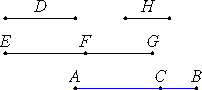# Proposition 48

To find the first binomial line.
X.28,Lemma1

Set out two numbers AC and CB such that the sum of them AB has to BC the ratio which a square number has to a square number, but does not have to CA the ratio which a square number has to a square number.

Set out any rational straight line D, and let EF be commensurable in length with D. Therefore EF is also rational.X.6,Cor.

Let it be contrived that the number BA is to AC as the square on EF is to the square on FG.

X.6

But AB has to AC the ratio which a number has to a number, therefore the square on EF also has to the square on FG the ratio which a number has to a number, so that the square on EF is commensurable with the square on FG.

X.9

And EF is rational, therefore FG is also rational. And, since BA does not have to AC the ratio which a square number has to a square number, neither, therefore, has the square on EF to the square on FG the ratio which a square number has to a square number. Therefore EF is incommensurable in length with FG.

X.36

Therefore EF and FG are rational straight lines commensurable in square only. Therefore EG is binomial.

I say that it is also a first binomial straight line.

Since the number BA is to AC as the square on EF is to the square on FG, while BA is greater than AC, therefore the square on EF is also greater than the square on FG.

Let then the sum of the squares on FG and H equal the square on EF.

V.19,Cor.

Now since BA is to AC as the square on EF is to the square on FG, therefore, in conversion, AB is to BC as the square on EF is to the square on H.

But AB has to BC the ratio which a square number has to a square number, therefore the square on EF also has to the square on H the ratio which a square number has to a square number.

X.9

Therefore EF is commensurable in length with H. Therefore the square on EF is greater than the square on FG by the square on a straight line commensurable with EF.

And EF and FG are rational, and EF is commensurable in length with D.

Therefore EF is a first binomial straight line.

Q.E.D.

## Guide

This proposition is not used in the rest of the Elements.